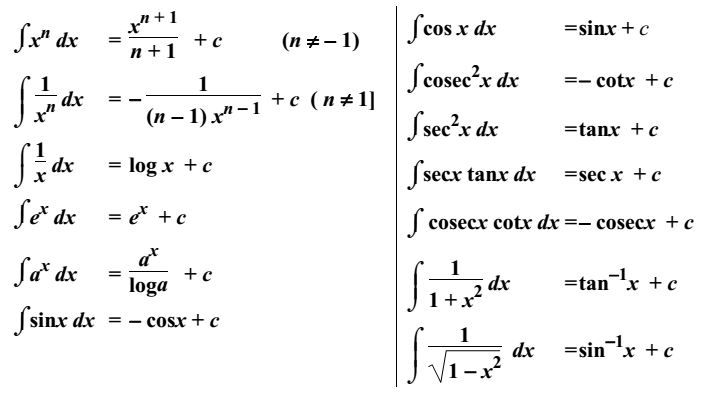# Integration

Integration is the inverse process of differentiation. A function F(x) is called an anti derivative or integral of a function f(x) on an interval I if

F′(x) = f(x) for every value of x in I

If the derivative of a function F(x) w.r.t. x is f(x), then the integral of f(x) w.r.t. x is F(x).

∫ f(x) dx = F(x) + C

The function f(x) is called Integrand. The variable x in dx is called variable of integration or integrator. The process of finding the integral is called integration.

C is called the constant of integration. Since C is arbitrary, ∫ f(x) dx is called the indefinite integral.

### Integration Formulae